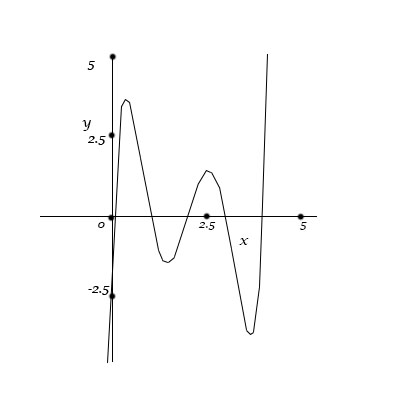# Refer to the graph of y=f(x) (a 5th degree polynomial) below. Exactly one of the following...

## Question:

Refer to the graph of {eq}y=f(x) {/eq} (a {eq}5^{th} {/eq} degree polynomial) below. Exactly one of the following statements is false. {eq}f''(0) < 0 {/eq}

{eq}f(x) {/eq} is concave-up on at least two disjoint closed intervals.

{eq}f'(0) > 0 {/eq}

There are at least {eq}4 {/eq} critical numbers.

{eq}f'''(x) {/eq} is defined everywhere.

{eq}f(x) {/eq} is concave-down on at least three disjoint open intervals.

{eq}f^{(VIII)} = 0 {/eq}

There are at least {eq}2 {/eq} inflection points.

{eq}f''(x) {/eq} is defined everywhere.

{eq}f'(x) {/eq} is defined everywhere.## Concavity and Inflection Points

A function is concave up if its second derivative is positive and a function is concave down if its second derivative is negative. The graph of a concave up function resembles the curve of a cup or a smile. The graph of a concave down function resembles the curve of a hill or a frown. An inflection point is a point where a function changes concavity - from concave up to concave down or from concave down to concave up

• We can see from the graph that {eq}f''(0)<0 {/eq} since the function is concave down on the interval {eq}(-\infty, 1] {/eq} where the right endpoint of the interval is a good estimate of the value.
• {eq}f'(0)>0 {/eq} since the function is increasing at {eq}x=0 {/eq}
• There are at least 4 critical numbers, at approximately {eq}x=0.5, 1.5, 2.5, 3.5 {/eq} , where the local maxima and minima occur.
• {eq}f'''(x) {/eq} is defined everywhere, since polynomials have derivatives of every order defined everywhere.
• {eq}f(x) {/eq} is concave-down on at least three disjoint open intervals since the function is concave down on {eq}(-\infty, 1)\cup(2,3)\cup(4,5) {/eq}
• The eighth derivative of the function is zero since every {eq}n {/eq}th order derivative of a polynomial where {eq}n {/eq} is larger than the degree of the polynomial is zero.
• There are inflection points at about {eq}x=1,2,3,4 {/eq} where the function changes concavity, so there are at least 2 inflection points.
• {eq}f''(x) {/eq} and {eq}f'(x) {/eq} are defined everywhere, since polynomials have derivatives of every order defined everywhere.

So the false statement is {eq}f(x) {/eq} is concave-up on at least two disjoint closed intervals since the function is concave up on {eq}(1,2)\cup(3,4) {/eq} (where the endpoints are estimated based on the appearance of the graph) , which are disjoint open intervals.